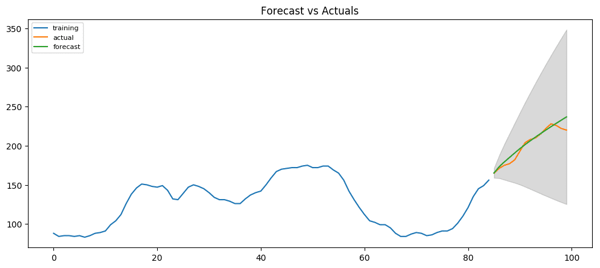Trusted answers to developer questions
Trusted Answers to Developer Questions

Related Tags

machine learning
arima
series
forecasting

# What is an ARIMA model?Educative Answers Team

Grokking Modern System Design Interview for Engineers & Managers

Ace your System Design Interview and take your career to the next level. Learn to handle the design of applications like Netflix, Quora, Facebook, Uber, and many more in a 45-min interview. Learn the RESHADED framework for architecting web-scale applications by determining requirements, constraints, and assumptions before diving into a step-by-step design process.

ARIMA is an acronym that stands for AutoRegressive Integrated Moving Average. The ARIMA model allows us to forecast a time series using the series’ past values.

A time series is a collection of data points collected at constant time intervals. Time series are used to forecast future values based on previous values.

Stationary Time series: A stationary time series is one whose statistical properties (mean, variance, autocorrelation, etc). are all constant over time. A non-stationary series is one whose statistical properties change over time.

An ARIMA model is characterized by 3 terms: $p, d, q$

• $p$ is the order of the AR (auto-regressive) part of the model term.

• $q$ is the order of the MA (moving average) term.

• $d$ is the number of differencing (i.e., the number of past time points to subtract from the current value) required to make the time series stationary.

The values for $p$ and $q$ can be determined using two plots:

• Autocorrelation function (ACF): A measure of the correlation between the time series and a lagged version of the time series. We can use ACF to determine the optimal number of MA ($q$) terms.

• Partial autocorrelation function (PACF): This measures the correlation between the time series and a lagged version of the time series after eliminating the variations already explained by the intervening comparisons. We can use PACF to determine the optimal number of terms ($q$) to use in the AR model.An example of a time series prediction plotted with the actual output. Image credits: Selva Prabhakaran (Machine Learning Plus)

## Non-stationary time series

For a non-stationary time series, the series has to be first transformed into a stationary series. The ARIMA model generally fits the non-stationary time series, based on the ARIMA model, with a differencing process that effectively transforms the non-stationary data into a stationary one. SARIMA models, which combine seasonal differencing with an ARIMA model, are used for time-series data modeling with periodic characteristics.

RELATED TAGS

machine learning
arima
series
forecasting